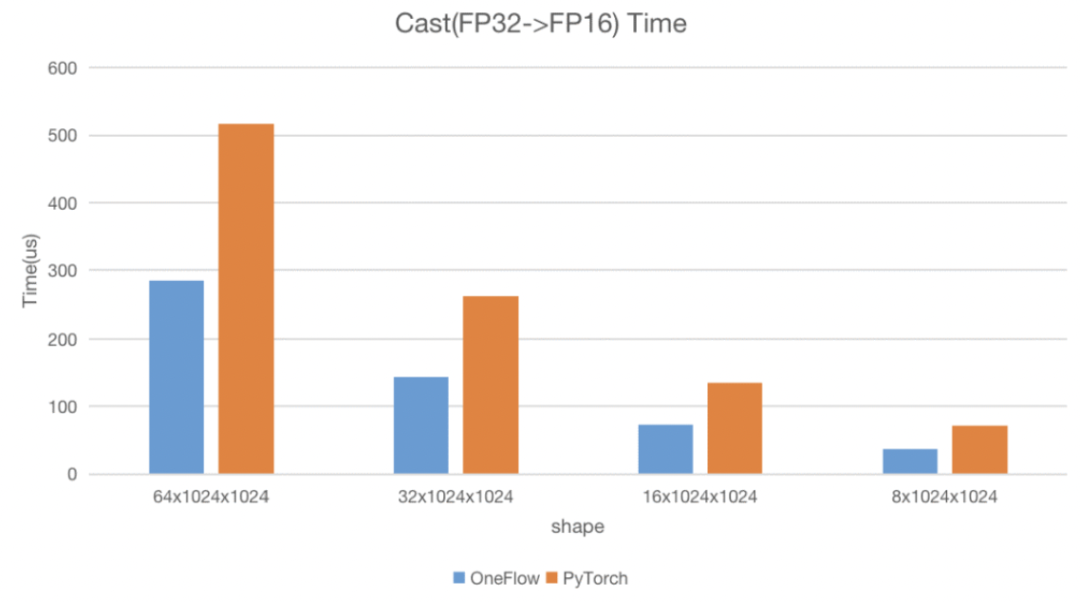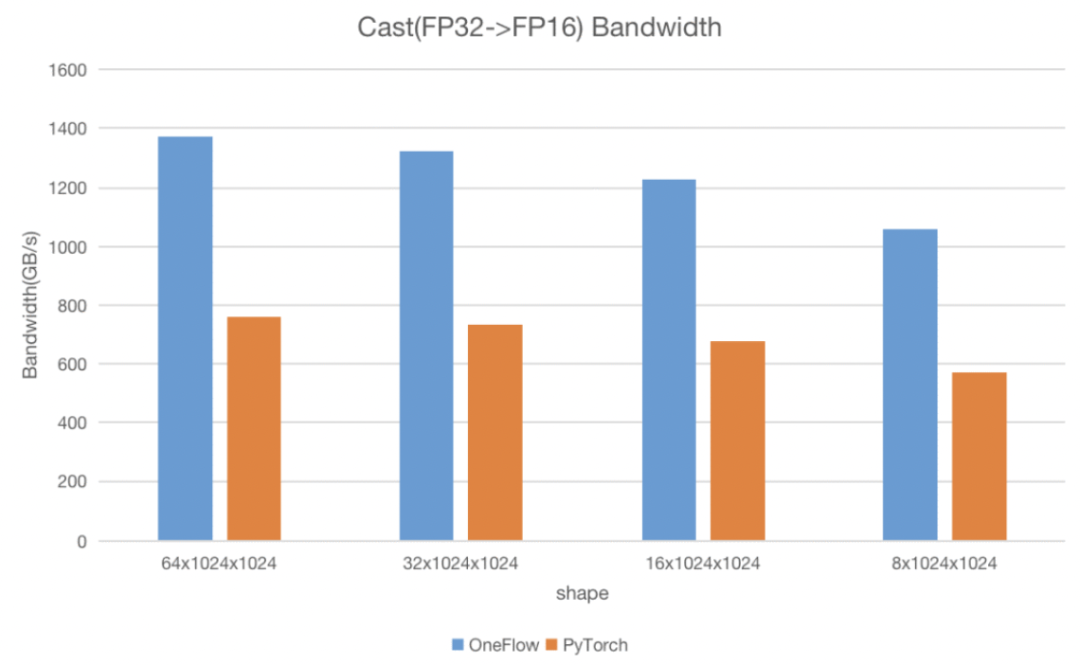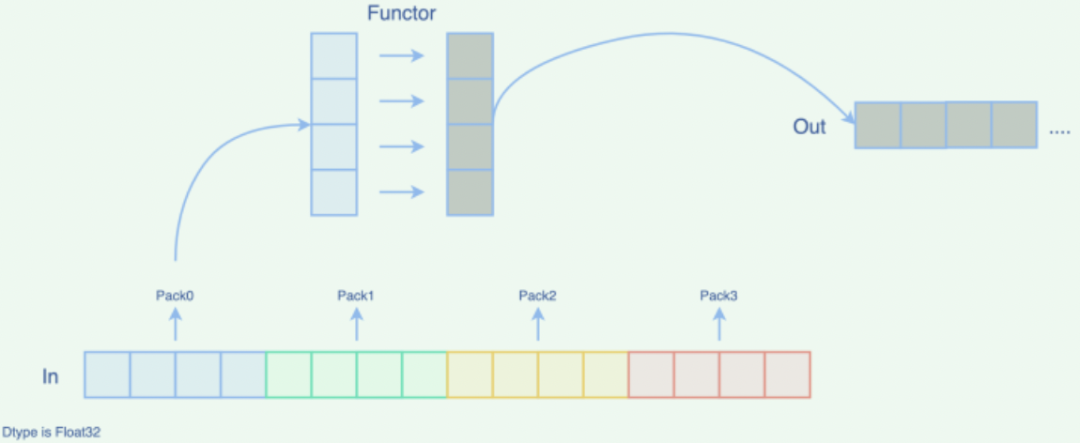> 文档中心 > 高效、易用、可拓展我全都要：OneFlow CUDA Elementwise模板库的设计优化思路

# 高效、易用、可拓展我全都要：OneFlow CUDA Elementwise模板库的设计优化思路``// Write ReLU Functor. templatestruct ReluFunctor {  OF_DEVICE_FUNC T operator()(T x) const {    const T zero_val = static_cast(0);     return (x > zero_val) ? x : zero_val;   }};// Use CUDA Elementwise Template. OF_CUDA_CHECK((cuda::elementwise::Unary(ReluFunctor(), elem_cnt, dx->mut_dptr(),                                        x->dptr(), ctx->stream()->As()->cuda_stream())));``1

• 主流架构里，每个 Block 最大寄存器数量是 64 K

• 每个线程所能使用的最大寄存器数量是 255 个

``constexpr int kBlockSize = 256constexpr int kNumWaves = 32;inline cudaError_t GetNumBlocks(int64_t n, int* num_blocks) {  ...  /*  n: The number of the elements.   sm_count: The number of the SM.   tpm: The maximum resident threads in per multiprocessor.   */  *num_blocks = std::max(1, std::min((n + kBlockSize - 1) / kBlockSize,                                                   sm_count * tpm / kBlockSize * kNumWaves));  return cudaSuccess;}``
• 线程块最小个数为1

• 线程块最大个数是从 处理所有元素所需最小的线程总数 和 wave 数目*GPU 一次可以调度 SM 数量 * 每个 SM 最大 block 数 中取最小值，这里我们的 wave 数目设置为固定32大小

2

``template __global__ void ker_layer_norm(T *ln_res, T *vars, T *means, const T *inp,                               const T *scale, const T *bias, int hidden_size) {  // step 0. compute local sum  float l_sum = 0;  float l_square_sum = 0;  const float4 *inp_f4 = (const float4 *)inp + blockIdx.x * hidden_size; // use float4  for (uint idx = threadIdx.x; idx < hidden_size; idx += blockDim.x) {    float4 val = inp_f4[idx];    ...  }}``

``templatestruct GetPackType {  using type = typename std::aligned_storage::type;};templateusing PackType = typename GetPackType::type;``

``templateunion Pack {  static_assert(sizeof(PackType) == sizeof(T) * pack_size, "");  __device__ Pack() {    // do nothing  }  PackType storage;  T elem[pack_size];};``

CUDA 里最大支持128 bit 的 pack 大小，而在浮点数据类型中，最小的类型（half）大小为16 bit，最多能把128 / 16=8 个 half 数据 pack 到一起，因此我们设置了这两个常量，kMaxPackBytes 表示 pack 最大字节数，kMaxPackSize 表示 pack 数据的最大个数：

``constexpr int kMaxPackBytes = 128 / 8;constexpr int kMaxPackSize = 8;``

3

``Unary/Binary/Ternary  -> xxxFactory     -> GenericLauncher::Launch       -> ApplyGeneric(CUDA Kernel)``

ApplyGeneric 这个 CUDA Kernel 中所做的主要工作是：

• 根据参数创建一个 functor

• 进入循环，针对打包（pack）后的数据，调用 ApplyPack 函数，每调用一次 ApplyPack，就处理一批 pack 后的数据

• 当最后存在元素个数不能被 pack_size 整除的情况时，需要让线程处理下尾部剩余元素

``template__global__ void __launch_bounds__(kBlockSize)    ApplyGeneric(FactoryT factory, int64_t n_pack, PackType* pack_r,                 const PackType*... pack_in, int64_t n_tail, R* tail_r,                 const IN*... tail_in) {  auto functor = factory();  const int global_tid = blockIdx.x * kBlockSize + threadIdx.x;  for (int64_t i = global_tid; i < n_pack; i += blockDim.x * gridDim.x) {    pack_r[i] = ApplyPack(        functor, (FetchPack(pack_in + i).elem)...);  }  if (tail && global_tid < n_tail) { tail_r[global_tid] = functor((tail_in[global_tid])...); }}``

ApplyPack函数定义如下，它对一个 pack 内的元素做了个循环，对 elem 数组中的每个元素调用 functor ，得到输出结果并返回：

``template__device__    typename std::enable_if<HasApply2::value == false, PackType>::type    ApplyPack(const FunctorT& functor, const IN... in[pack_size]) {  Pack ret;#pragma unroll  for (int j = 0; j < pack_size; ++j) { ret.elem[j] = functor((in[j])...); }  return ret.storage;}``4

``template__device__ typename std::enable_if<HasApply2::value == true && pack_size % 2 == 0,                                   PackType>::typeApplyPack(const FunctorT& functor, const IN... in[pack_size]) {  Pack ret;#pragma unroll  for (int j = 0; j < pack_size; j += 2) { functor.Apply2(ret.elem + j, (in + j)...); }  return ret.storage;}``

``templatestruct CastFunctor<half, From, typename std::enable_if<!std::is_same::value>::type> {  ...  __device__ void Apply2(half* to, const From* from) const {    float2 f2;    f2.x = static_cast(from);    f2.y = static_cast(from);    *reinterpret_cast(to) = __float22half2_rn(f2);  }};``

5

``templatestruct SimpleFactory {  explicit SimpleFactory(FunctorT functor) : tpl(functor) {}  __device__ FunctorT operator()() const { return tpl; } private:  FunctorT tpl;};templateinline cudaError_t UnaryWithFactory(FactoryT factory, int64_t n, R* r, const A* a,                                    cudaStream_t stream) {  return GenericLauncher::Launch(factory, n, r, a, stream);}templateinline cudaError_t Unary(FunctorT functor, int64_t n, R* r, const A* a, cudaStream_t stream) {  return UnaryWithFactory(SimpleFactory(functor), n, r, a, stream);}// BinaryWithFactory TernaryWithFactory ... // Binary Ternary ...``

• 性能够高，应用这套 Elementwise 模板的算子都能打满机器的带宽，速度也够快。

• 开发效率高，开发人员可以不用过分关注 CUDA 逻辑及相关优化手段，只需要编写计算逻辑即可。

• 可扩展性强，目前这套模板支持了一元，二元，三元操作。若今后有需求拓展，支持更多输入时，只需要仿照编写对应的工厂即可。

• 动态调度的“诅咒”

• OneFlow四大训练赛题等你来战

• 用OneFlow实现ISBI细胞分割任务

• CUDA优化之LayerNorm性能优化实践

• Credit-based Flow Control的前世今生

• 计算机史最疯狂一幕：“蓝色巨人”奋身一跃